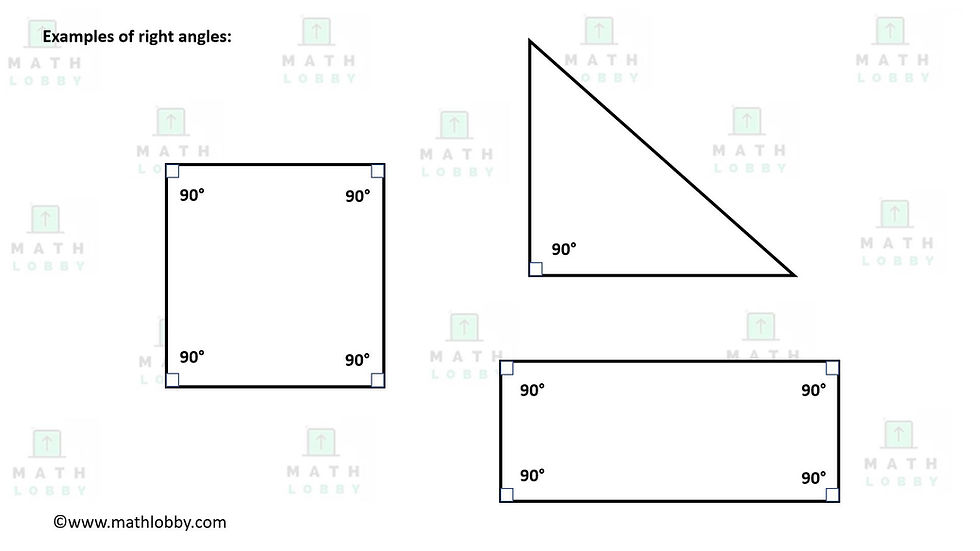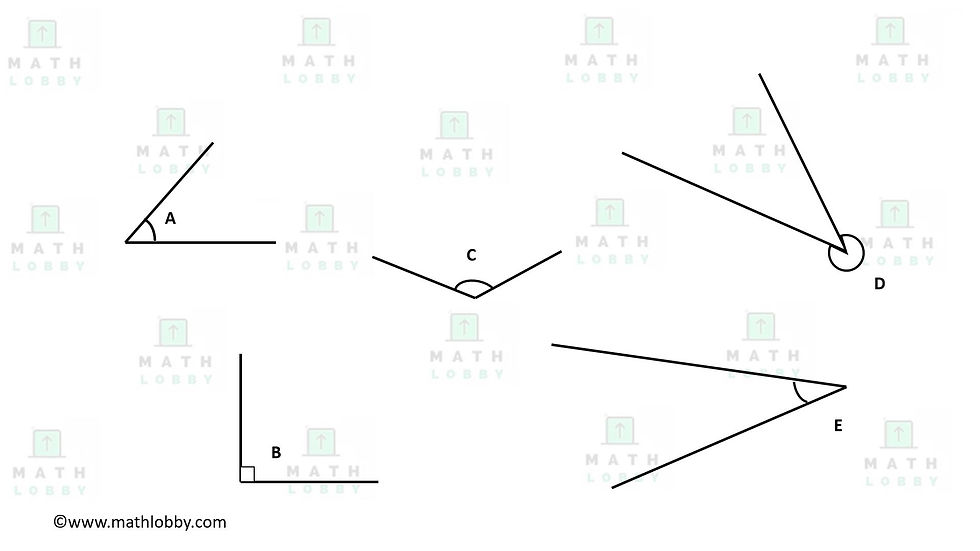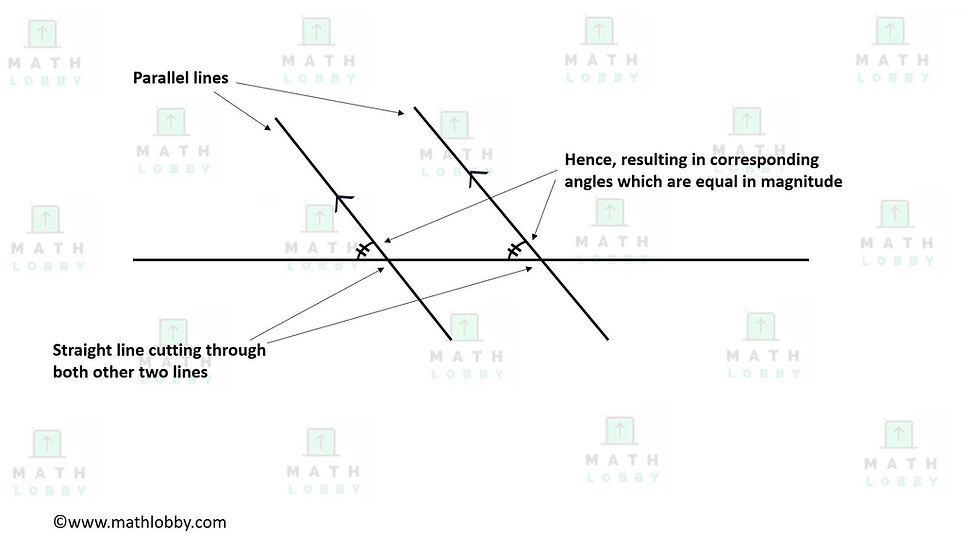top of page
Search

# Angles in Geometry

Updated: Jun 22, 2021

Dear Secondary Math students, Math Lobby will be covering Angles in Geometry today. Let’s begin!

In this note, you will learn:

1) What are acute, right, obtuse and reflex angles?

2) Vertically opposite angles, angles on a straight line and angles at a point

3) Angles formed by two parallel lines and a transversal: corresponding angles, alternate angles and interior angles

### 1) What are acute, right, obtuse and reflex angles?

In secondary mathematics, we will encounter many questions that involve angles when dealing with geometry and it is important to know what names are given to specific types of angles. Let’s take a look below!

Acute angles – Any angle that is LESS than 90°Right angles – Any angle that is exactly 90°Obtuse angles – Any angle that is in between 90° and 180°Reflex angles – Any angle that is between 180° and 360°Question time!In the diagram above, can you identify which angle belongs to which category?

### 2) Vertically opposite angles, angles on a straight line and angles at a point

Now that you know the types of angles that exist, there are some rules in geometry that is beneficial in helping us to identify unknown angles with the help of those that are known.

The first rule that we will be going through is called vertically opposite angles. Vertically opposite angles are the angles opposite each other when two straight lines crosses each other. “Vertical” in this context does not mean that the top is exactly inline with its bottom, but just means that the angles share the same vertex or corner point.Next, angles that are on a straight line will always sum up to 180°, and angles at a point will always sum up to 360°.In the diagram above, we can see that even if we only have certain angles that are known/provided, we still can identify and apply these rules to solve for the unknown angles.

### 3) Angles formed by two parallel lines and a transversal: corresponding angles, alternate angles and interior angles

Apart from vertically opposite angles, there are a few more rules that involve parallel lines and a transversal, which is essentially the cutting of a system of lines. The first one we will be talking about are called corresponding angles. Corresponding angles are basically two angles in a similar relative position at each intersection when a straight line crosses the other two lines. If the two lines are parallel, the angles will be equal.Alternate angles, on the other hand, are angles that are equal in magnitude, in opposite positions relative to the transversal intersecting two lines, which are parallel.By definition, angles that lie in between the two parallel lines intersected by the transversal are known as interior angles. You can see it in the diagram below for a better visualization*Note: Another definition of “interior angles” involves the concept and understanding of polygons, which will be covered in the next article!

Now, it is time to put on your thinking caps and try out questions on your own!Given that ∠BAC = 50°, ∠ACF = 105° and ∠GFH = 30°, find

i) ∠FHE

ii) ∠GAC

iii) ∠AGC

And that’s all we have for today, students! Math Lobby hopes that you have a clear understanding of the different types of angles and rules in geometry after what we went through today, and if you have any questions or inquiries, feel free to contact Math Lobby on our website, Facebook or Instagram page! As always: Work hard, stay motivated and we wish all students a successful and enjoyable journey with Math Lobby!

If you want to receive daily Secondary Math Tips from us,

FOLLOW our Instagram page at https://www.instagram.com/mathlobbymotivation/